Courses

# Test Level 3: Electrostatics

## 30 Questions MCQ Test Physics Class 12 | Test Level 3: Electrostatics

Description
This mock test of Test Level 3: Electrostatics for Class 12 helps you for every Class 12 entrance exam. This contains 30 Multiple Choice Questions for Class 12 Test Level 3: Electrostatics (mcq) to study with solutions a complete question bank. The solved questions answers in this Test Level 3: Electrostatics quiz give you a good mix of easy questions and tough questions. Class 12 students definitely take this Test Level 3: Electrostatics exercise for a better result in the exam. You can find other Test Level 3: Electrostatics extra questions, long questions & short questions for Class 12 on EduRev as well by searching above.
QUESTION: 1

### Three positive charges of equal value q are placed at the vertices of an equilateral triangle. The resulting lines of force should be sketched as in

Solution:

Carefully observe each property of electric field lines in the above options.

QUESTION: 2

### What will be the value of a charge q, such that when it is placed at the centre of two equal and like charges Q, the three charges are in equilibrium.

Solution:

If we see all the forces acting upon the charge Q due to other charge Q at distance r and other charge q at distance r/2, we get
kQQ/r2 + kQ(q)/(r/2)2
This results
Q2 / r2  = -4Qq / r2
Thus we get q = -Q/4

QUESTION: 3

### A charged particle having some mass is resting in equilibrium at a height H above the centre of a uniformly charged non-conducting horizontal ring of radius R. The force of gravity acts downwards. The equilibrium of the particle will be stable -

Solution:

electric field due to non-conducting ring along its axis is given by,
E=kqz/(Z2+r2)3/2
where z is the separation between point of observation to the centre of the ring, q is the charge on the ring and r is the radius of the ring.
We know, electric field will be maximum only when z = R/√2, meaning at this point the value of force must be maximum. and if we increase the value of z from R/√2 it will decrease and if we decrease the value of z from R/√2 , it will increase.
condition of stable equilibrium,
A small vertical displacement upwards should cause the resultant force on the particle to be downwards, to return it or a small vertical displacement downward should cause the resultant force on the particle to be downward.
hence, at z > R/√2 or, h > R/√2 system will be in stable equilibrium.

QUESTION: 4

In space of horizontal EF(E = (mg)/q) exist as shown in figure and a mass m attached at the end of a light rod. If mass m is released from the position shown in figure find the angular velocity of the rod when it passes through the bottom most position.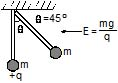Solution:

According to the work energy theorem we have
We​+Wg​=(1​/2)mv2
We have work done by electrostatic force as
We​=qElsinθ
and work done by the gravitational force as
Wg​=mg(l−lcosθ)
Thus we get
qElsinθ+mg(l−lcosθ)=(1/2)​mv2
Thus we get
mgsinθ+mgl−mglcosθ=(1/2)​mv2
as θ=45o, we get
mgl=(1/2)​mv2
also as v=ωl
we get
ω=√2g/​​l

QUESTION: 5

Find the force experienced by the semicircular rod charged with a charge q, placed as shown in figure. Radius of the wire is R and the infinitely long line of charge with linear density l is passing through its centre and perpendicular to the plane of wire.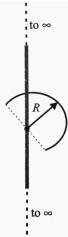Solution:

Fnet​=∫dqEcosθ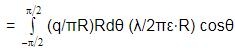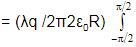cosθdθ=(λq/2π2ε0​R) ​[sinθ]−π/2π/2
= (λq/2π2ε0​R) ​[1−(−1)]= λq​/π2ε0​​R

QUESTION: 6

A wheel having mass m has charges +q and –q on diametrically opposite points. It remains in equilibrium on a rough inclined plane in the presence of uniform vertical electric field E =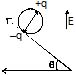Solution: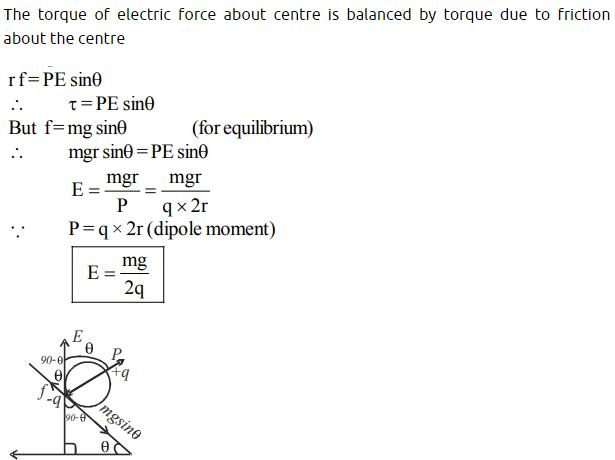*Multiple options can be correct
QUESTION: 7

Select the correct alternative :

Solution:

A.Statement A is wrong.
B.m= m0​​/√(1− v2/c2) ​​
specific charge q/m=q/m0√(1​−(v2/C2))​​
so, as v increases the specific charge decreases
C. None of the fundamental particles that form the Standard Model have charge without mass. The Standard Model describes all energy and matter that makes up the universe, except gravitation. And gravitation does not have charge, it has mass.
D.Yes. Repulsion is observed only when two bodies have like charges that means the bodies must be charged. Therefore, repulsion is sure test for electrification than attraction.

*Multiple options can be correct
QUESTION: 8

Two equal negative charges –q are fixed at the point (0, a) and (0,– a) on the y-axis. A charge +Q is released from rest at the point (2a, 0) on the x-axis. The charge Q will :

Solution:

The net force is given as,
Fn​=2Fcosθ
=2×(1/4πε0)​( −qQ /a2+x2 ​)×[x/​(a2+x2)1/2]
Thus, the restoring force is not linear, So, the motion will not be simple harmonic motion but the motion will be oscillatory.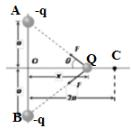*Multiple options can be correct
QUESTION: 9

Mid way between the two equal and similar charges, we placed the third equal and similar charge. Which of the following statements is correct, concerned to the equilibrium along the line joining the charges ?

Solution: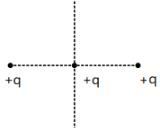The charge in the middle experiences force along the line. The equilibrium is stable along the line connecting charges while The equilibrium is unstable along the line perpendicular to the line of charges
∴ Only option B is correct (given consider equilibrium only along line joining charges).

*Multiple options can be correct
QUESTION: 10

A negative point charge placed at the point A is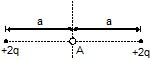Solution:

If the potential energy of the system is minimum, it will be stable equilibrium, i.e , d2U/dx2​>0 and when potential energy is maximum then it will be unstable equilibrium, i.e, d2U/dx2<0.
As along y direction no electric field, potential energy is minimum and it will be stable equilibrium along y-axis.
Along x-axis potential energy is maximum due to all charges situated along x-axis.so it will be unstable equilibrium.

*Multiple options can be correct
QUESTION: 11

Two fixed charges 4Q (positive) and Q (negative) are located at A and B, the distance AB being 3 m.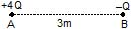Solution:

(A) electric field due to positive charge is away from it and electric field due to negative charge is towards it so there cannot be a point on AB where electric field can be zero
(D) negative charge will experience a repulsion force due to −ve charge and attraction force due to +ve charge, so if pushed away from charges then a net attractive force and if pushed towards AB then a net repulsive force will be there so there will be oscillations.

*Multiple options can be correct
QUESTION: 12

Select the correct statement : (Only force on a particle is due to electric field)

Solution:

If field is straight then the charge moves along the straight line .if the fielss is a curve then the electrostatic force is not sufficient to change the direction along the curved field line .

QUESTION: 13

Consider a conductor with a spherical cavity in it. A point charge q0 is placed at the centre of cavity and a point charge Q is placed outside conductor.

Statement-1 : Total charge induced on cavity wall is equal and opposite to the charge inside.
Statement-2 : If cavity is surrounded by a Gaussian surface, where all parts of Gaussian surface are
located inside the conductor,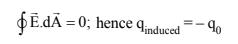Solution:
QUESTION: 14

The figure shows a nonconducting ring which has positive and negative charge non uniformly distributed on it such that the total charge is zero. Which of the following statements is true ?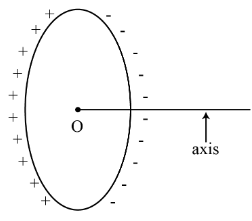Solution: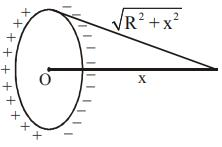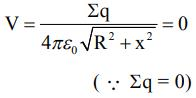There for the potential at all the points on the axis will be zero.

*Multiple options can be correct
QUESTION: 15

If we use permittivity e, resistance R, gravitational constant G and voltage V as fundamental physical quantities, then -

Solution: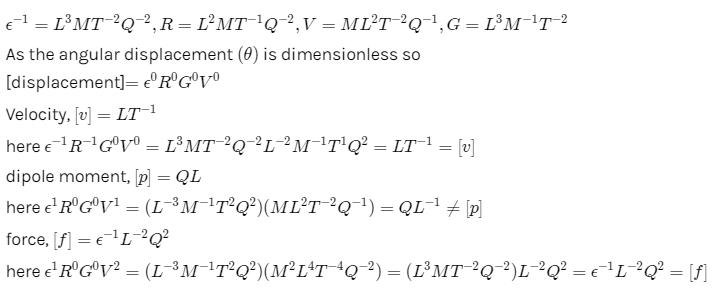*Multiple options can be correct
QUESTION: 16

A particle of mass m and charge q is thrown in a region where uniform gravitational field and electric field are present. The path of particle

Solution:

The acceleration due to gravity is insignificant compared with the acceleration caused by the electric field, so the gravitational field can be ignored. Hence, when a particle of mass m and charge q is thrown in a region where uniform gravitational field and electric field are present, before entering the electric field, the charge follows a straight line path (since, no net force due to any of the field). As soon as it enters the field, the charge begins to follow a parabolic path (since, constant force is always in the same direction). As soon as it leaves the field, the charge follows a straight line path  (since, no net force due to any of the field). The path of a particle may be straight line or may be parabola.

*Multiple options can be correct
QUESTION: 17

Statement - 1 : A positive point charge initially at rest in a uniform electric field starts moving along electric lines of forces. (Neglect all other forces except electric forces)

Statement - 2 : Electric lines of force represents path of charged particle which is released from rest in it.

Solution:
QUESTION: 18

Two equal negative charges are fixed at the points [0, a] and [0, –a] on the y-axis. A positive charge Q is released from rest at the points [2a, 0] on the x-axis. The charge Q will -

Solution:
QUESTION: 19

Two small balls, each having equal positive charge Q are suspended by two insulating strings of equal length L from a hook fixed to a stand. If the whole set-up is transferred to a satellite in orbit around the earth,the tension in each string is equal to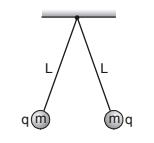Solution:

A satellite is in a state of free fall & hence weightlessness. Thus only electric force is responsible for the Tension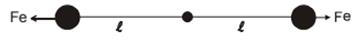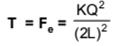QUESTION: 20

An electric dipole is placed at an angle of 30o to a non-uniform electric field. The dipole will experience

Solution:

The dipole will have some distance along the electric field, so, option (1) is correct.

QUESTION: 21

Two point charges +8q and –2q are located at x = 0 and x = L respectively. The location of a point onthe x axis at which the net electric field due to these two point charges is zero is:

Solution:

Suppose that at point B, where net electric field is zero due to charges 8q and 2q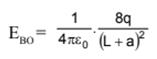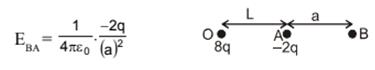According to conditional EBO+ EBA = 0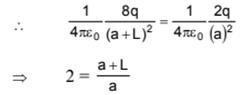So a = L

Thus, at distance 2L from origin, net electric field will be zero

QUESTION: 22

The figure shows the electric field lines in the vicinity of two point charges. Which one of the followingstatements concerning this situation is true?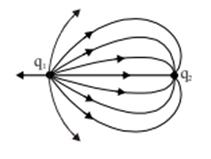Solution:
QUESTION: 23

Four charges are placed each at a distance a from origin. The dipole moment of configuration is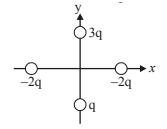Solution:

This question can be solved easily , if you have some knowledge about resolution of vectors.
all four charges are placed , each at a distance 'a ' from the origin.
it means distance between two charges = √{a² + a² } = √2a
We also know, dipole moment is the system of two equal magnitude but opposite nature charges . so, we have to divide the charges as shown in the figure for making dipoles .
Hence, there are three dipoles formed.
Let P = q(√2a)
then, P₁ = P , P₂ = P and P₃ = 2P {as shown in figure.}
now resolve the vectors P₁ ,P₂ and P₃ .
as shown in figure,
vertical components of P₁ and P₂ is cancelled .
and horizontal components of P₁ and P₂ is cancelled by horizontal component of P₃ .
and rest part of dipole = vertical component of P₃ = 2Psin45° j ['j' shows direction of net dipole moment]
hence, net dipole moment = 2q(√2a) × 1/√2 j
= 2qa j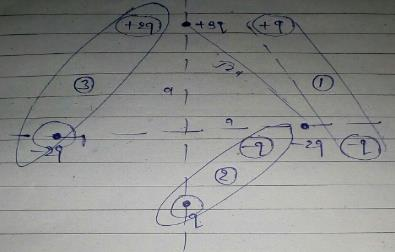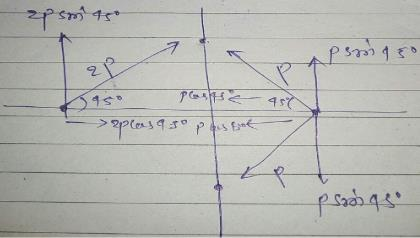QUESTION: 24

Figure shows the electric field lines around an electric dipole. Which of the arrows best represents the electric field at point P?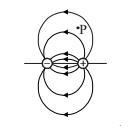Solution:

Tangent to the electric field line represents the direction of electric field at that point.

QUESTION: 25

A uniform electric field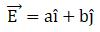, intersects a surface of area A. What is the flux through this area if thesurface lies in the yz plane?

Solution: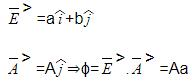QUESTION: 26

If 109 electrons move out of a body to another body every second, how much time is approximately required to get a total charge of 1 C on the other body?

Solution:

we know that 1 colomb = 6.242 x 1018 electron
given 109 electron take 1 sec
⇒ 10electron → 1 sec
⇒ 1 electron →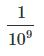secs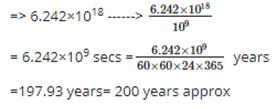QUESTION: 27

A uniform line charge with linear density λ lies along the y-axis. What flux crosses a spherical surface centered at the origin with r = R

Solution:
QUESTION: 28

Electric lines of force never intersect each other because

Solution:

Electric lines of force never intersect each other because at the point of intersection, two tangents can be drawn to the two lines of force. This means two direction of electric field at the point of intersection, which is not possible.

QUESTION: 29

A neutral point lies at the center of the line joining the charges when

Solution:

Neutral point due to a system of two like point charges For this case neutral point is obtained at an internal point along the line joining two like charges.Here neutral point lies outside the line joining two unlike charges and also it lies nearer to the charge which is smaller in magnitude.

QUESTION: 30

The dimensions of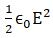where [∈0 : permittivity of free space ; E: electric field]

Solution:

Dimensions of permittivity of free space ∈= [q2/Fr2] and
By definition of Electric Field Intensity; [E] =[ F/q]